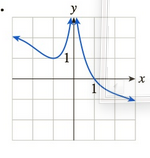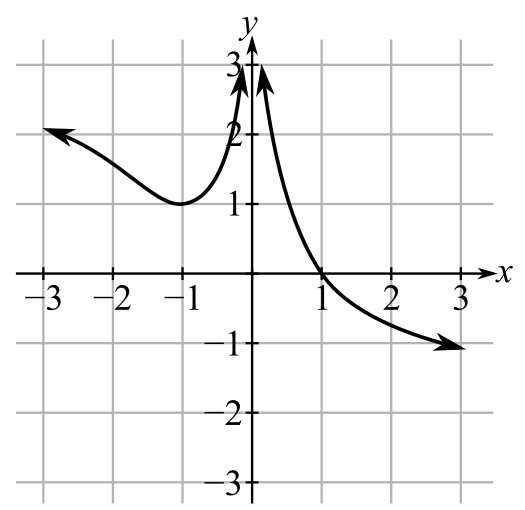Chapter 10.2, Problem 11EFinite Mathematics and Applied Cal...

7th Edition
Stefan Waner + 1 other
ISBN: 9781337274203

Solutions

Chapter
SectionFinite Mathematics and Applied Cal...

7th Edition
Stefan Waner + 1 other
ISBN: 9781337274203
Textbook Problem

In Exercises 1–14 the graph of a function f is given. Determine whether f is continuous on its domain. If it is not continuous on its domain, say why. [HINT: See Quick Examples 1–4.]To determine

Whether the function f is continuous on its domain and give reason if it is not continuous. The graph of function f is as follows:Explanation

Given Information:

The provided graph is:

Consider the provided graph:

The domain of f consists of all real numbers except 0

Still sussing out bartleby?

Check out a sample textbook solution.

See a sample solution

The Solution to Your Study Problems

Bartleby provides explanations to thousands of textbook problems written by our experts, many with advanced degrees!

Get Started

If 2x g(x) x4 x2 + 2 for all x, evaluate limx1g(x)

Single Variable Calculus: Early Transcendentals, Volume I

Domain Find the domain of the expression. 7. 4x2 10x + 3

Precalculus: Mathematics for Calculus (Standalone Book)

In Exercises 7-12, solve for y in terms of x. x=2y13

Calculus: An Applied Approach (MindTap Course List)

In Exercises 1-6, simplify the expression. 3. 12t2+12t+34t21

Applied Calculus for the Managerial, Life, and Social Sciences: A Brief Approach

Evaluate the indefinite integral. sin2x1+cos2xdx

Single Variable Calculus: Early Transcendentals

limx0cscx= a) 0 b) c) d) does not exist

Study Guide for Stewart's Single Variable Calculus: Early Transcendentals, 8th

In Exercises 110, evaluate the expression. P(10,4)C(12,6)

Finite Mathematics for the Managerial, Life, and Social Sciences